# If Else Statements in C Language

## If Else statements in C Language with Examples

In this article, I will discuss If Else Statements in C Language with Examples, i.e., how if and if-else block gets executed with the help of syntax, flow chart, and multiple examples. Please read our previous article discussing the basics of Control Statements in C. Before understanding if-else statements, let us understand the Selection Statements in C Language.

##### What are Selection Statements in C?

Selection statements allow us to control the flow of program execution based on the outcome of an expression or state of a variable known during run time. It executes different sections of code depending on a specific condition or the value of the variable. Selection statements can be divided into the following categories:

• switch statements (Will be discussed in the next article)
##### If Block in C Programming Language:

It executes a block of instructions (one or more instructions) when the condition in the if block is true, and when the condition is false, it will skip the execution of the if block. Following is the syntax to use the if block.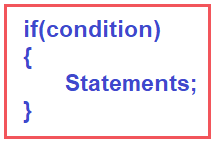##### Flow Chart of If Block:

Let us see how we will represent the execution flow of the if block using a flow chart. The oval symbol represents the starting point. A diamond shape represents the condition. Here, first, we need to check the condition. For every condition, there are two options, i.e., the condition is true, and the condition is false. That means two situations are there, i.e. TRUE and FALSE. Suppose the condition is TRUE; all statements are defined inside the block if it gets executed. And the statements we are representing in the flow chart with the help of a parallelogram symbol. After the execution of the statements, the control will come to an end. Suppose the condition is false; then, without executing any statement, it will end. For better understanding, please look at the diagram below, which represents the flow chart of the if conditional statement.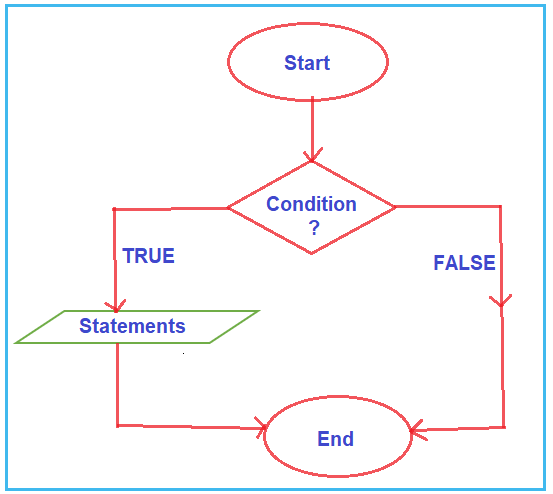Note: Here, the block of statements executes only when the condition is true. If the condition is false, it will skip the execution of the statements.

##### Example to Check Whether the Number is Greater than 10

Here, we will take the number from the user and then check whether that number is greater than 10 or not using the If Statement in C Language. The following program exactly does the same.

```#include<stdio.h>
int main()
{
int number;
printf("Enter a Number : ");
scanf("%d", &number);
if(number > 10)
{
printf("%d is greater than 10 \n", number);
printf("End of if block \n");
}

printf("End of Main Method");
return 0;
}
```

In the above program, inside the main method, we declare one integer variable, i.e., number. Then, we take the input from the user by using the scanf function and store it in the number variable.

After reading the input, we check whether the given number is greater than 10. If the number > 10, then the condition becomes true, and, in that case, we are executing the two statements that are present inside the block. If the condition is false, then the if block statements will be skipped, and the last printf statement will get executed.

For example,

1. If we take 15 as an input, 15  > 10 means the condition is true, then the if block statement gets executed.
2. If we take 5 as an input. 5 > 10 means the condition is false, then the if block statements will be skipped.

For a better understanding, please have a look at the image below.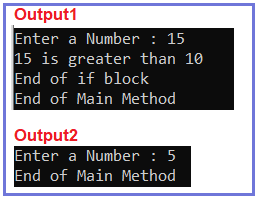##### If Statement without Curly Braces in C Language

In the declaration of the If block, if we do not specify statements using blocks ({}) nothing but curly braces, then only the first statement will be considered as the if block statement. To understand this point, please have a look at the below example.

```#include<stdio.h>
int main()
{
int number;
printf("Enter a Number : ");
scanf("%d", &number);
if(number > 10)
printf("%d is greater than 10 \n", number);
printf("End of Main Method");

return 0;
}
```

As you can see, in the above example, we have not specified the curly braces to define the if block. In this case, only the first statement will be considered the if block statement. The second statement will not be a part of the if block. For a better understanding, please have a look at the below image. The statement in red color will belong to the if block and the statement in the black color will not belong to the if block.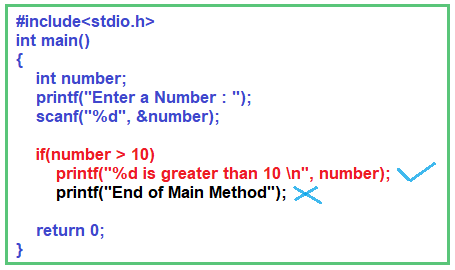So, when we execute the above program, the black statement will always be executed irrespective of the condition, as it is not part of the if block. The red statement is only executed when the If condition is true. For a better understanding, please have a look at the image below.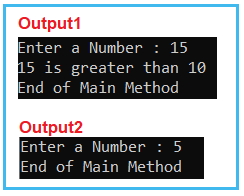##### If Else Block in Programming Language:

The If-Else block provides some optional information whenever the given condition is FALSE in the if block. That means if the condition is true, then the if block statements will execute, and if the condition is false, then the else block statement will execute. The following is the syntax to use the IF ELSE block in most programming languages.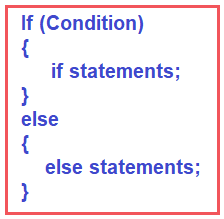Note: You need to remember only one block of statement, i.e., if block or else block will be executed at a time. So, if the condition is TRUE, the IF block statements get executed; if the condition is FALSE, the Else block statements get executed.

###### Is it mandatory to use the Else block?

No, it is not mandatory to use the Else block. It is an optional block. If you want to provide information when the condition fails, use this optional else block.

##### Flow Chart of If-Else Block:

The flow chart of the if-else block is almost similar to the if block. In this case, when the condition is true, the if block statements get executed, and when the condition is false, the else block statements get executed. For a better understanding, please have a look at the image below, which shows the flow chart of the if-else block.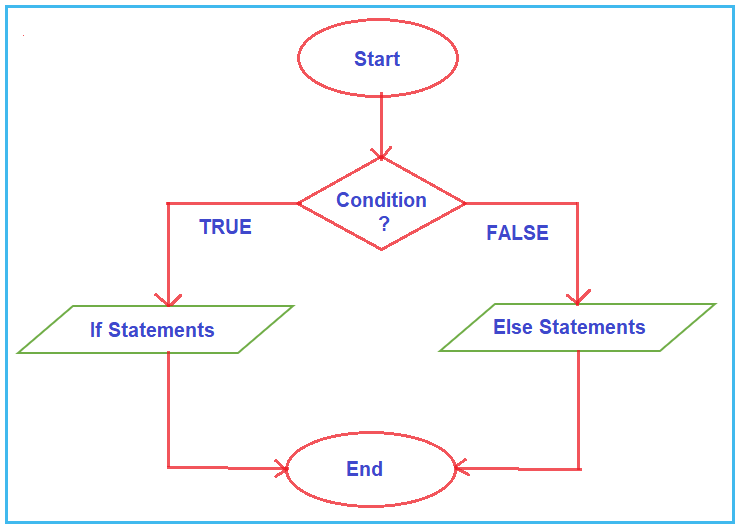Note: In C Programming Langauge, if and else are reserved words. The expressions or conditions specified in the if block can be a Relational or Boolean expression or condition that evaluates to a TRUE(1) or FALSE(0).

In C programming, if-else statements are a fundamental way to perform decision-making operations. They allow the program to execute certain parts of code depending on whether a condition is true or false. Now, let us see some examples to understand the if-else conditional statements.

##### Example to Check Whether a Number is Even or Odd.

Here, we will take the input number from the user and then check whether that number is even or odd using the if-else statement in C Language. The following program exactly does the same.

```#include<stdio.h>
int main()
{
int number;
printf("Enter a Number : ");
scanf("%d", &number);
if(number % 2 == 0)
{
printf("%d is an Even Number", number);
}
else
{
printf("%d is an Odd Number", number);
}
return 0;
}
```

In the above program, inside the main method, we declare one integer variable, i.e., number. Then, we read input from the user using the scan function and store the value in the address of the number variable. After reading the input, we check whether the given number is even or odd. An Even number is a number that is divisible by 2.

If number % 2 equals 0, then the if condition is true, and, in that case, we are printing a message that it is an even number, and if the condition is false, then we are printing a message that it is an odd number.

For example,

1. If we take 16 as an input, 16%2 == 0 means the condition is true, then the if block statement gets executed. The output will be 16 is an Even Number.
2. If we take 13 as an input, 13%2 == 0 means the condition is false, then the else block statements get executed. The output will be 13 is an Odd Number.

For a better understanding, please have a look at the image below.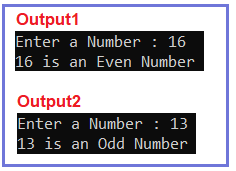##### If and Else Block without Curly Braces in C Programming Language

In the declaration of if block or else block, if we do not specify statements using blocks ({}) nothing but curly braces, then only the first statement will be considered the if block or else block statement. Let us understand this point with some examples. Please have a look at the below example.

```#include<stdio.h>
int main()
{
if(1 == 1)
printf("Hi\n");
else
printf("Hello\n");
printf("Bye\n");
return 0;
}
```

As you can see, we did not specify the curly braces in the above example while creating the if and else blocks. So, in this case, the first print statement will belong to the if block. After the else statement, we have two printf statements. The printf statement that prints the Hello message will only belong to the else block. The next print statement, which prints the message bye, will not belong to the else block. If you execute the above program, you will get the following output.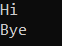An ERROR message will be displayed if we replace the Hello statement in the if block. The reason is that no more than one statement gets executed without braces. One statement will execute inside the if block. If we want to execute more than one statement, we should use braces, and all the statements will be inside the braces. For a better understanding, please have a look at the below example.

```#include<stdio.h>
int main()
{
if(1 == 1)
printf("Hi\n");
printf("Hello\n");
else
printf("Bye\n");
return 0;
}
```

Now, while compiling the code, you will get the following error.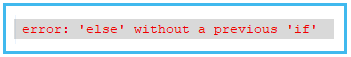Note: For every if condition statement, the else block is optional. But for every Else block, If block is compulsory. The purpose of the ‘if’ statement in a program is to allow multiple execution paths for varying user inputs, making it more interactive!